DLI DC3 Object Model Reference
20210819T152642Z
Renderer object
 object renderer/ Object representing renderer capabilities and functions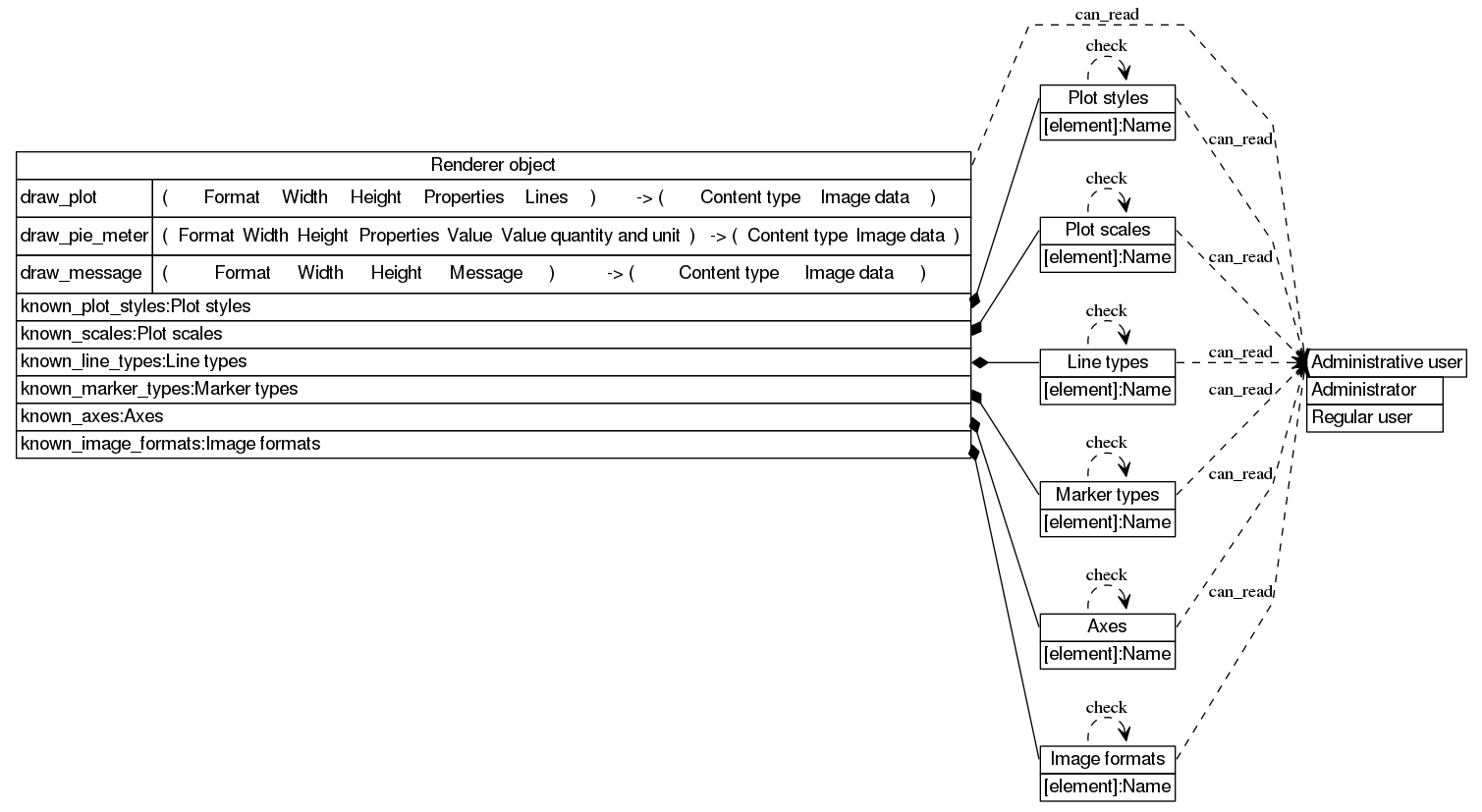Retrieving the value is denied if not administrative user

This object contains the following items:

Sample CLI write command:

```uom set renderer "{\"known_scales\":{\"linear\":\"linear\",\"log\":\"logarithmic\"},\"known_marker_types\":{\"circle\":\"Filled circle\",\"square\":\"Filled square\",\"otriangle\":\"Empty triangle\",\"osquare\":\"Empty square\",\"triangle\":\"Filled triangle\",\"rhombus\":\"Filled rhombus\",\"orhombus\":\"Empty rhombus\",\"plus\":\"Plus\",\"dot\":\"Dot\",\"rotriangle\":\"Empty inverse triangle\",\"rtriangle\":\"Filled inverse triangle\",\"ocircle\":\"Empty circle\",\"cross\":\"Cross\"},\"known_plot_styles\":{\"lines\":\"Lines only\",\"steps\":\"Step lines\",\"bezier\":\"Bezier smoothed lines\",\"linespoints\":\"Lines and markers\",\"impulses\":\"Vertical lines\",\"dots\":\"Small dots\",\"csplines\":\"Spline smoothed lines\",\"points\":\"Markers only\",\"boxes\":\"Boxes\",\"acsplines\":\"Approximated spline smoothed lines\"},\"known_line_types\":{\"dashed\":\"Dashed\",\"dotted\":\"Dotted\",\"solid\":\"Solid\"},\"known_image_formats\":{\"svg\":\"SVG\",\"gif\":\"GIF\",\"jpeg\":\"JPEG\",\"png\":\"PNG\"},\"known_axes\":{\"x2y2\":\"X2, Y2\",\"x2y1\":\"X2, Y1\",\"x1y1\":\"X1, Y1\",\"x1y2\":\"X1, Y2\"}}"
```

Sample `curl` read command:

```curl --digest -u admin:1234 -H "Accept: application/json" "http://192.168.0.100/restapi/renderer/"
```

# Draw a plot

 call renderer/draw_plot/ Generate an image with axes and data plotted on them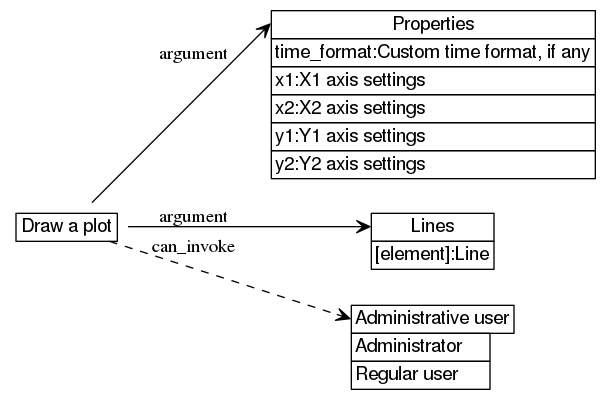Performing the function call is denied if not administrative user

The call accepts the following arguments:

1. Format: string
2. Width: number
3. Height: number
4. Properties: object "Properties"
5. Lines: array of "(string,array of "(number,None or Y)",object "Style",(object "X axis quantity and unit",object "Y axis quantity and unit"))"

The call returns the following results:

1. Content type: string
2. Image data: string

Sample `requests` invocation:

```print(requests.post('http://192.168.0.100/restapi/renderer/draw_plot/',auth=auth,headers=headers,json=<...>).json())
```

```'<binary data of type image/gif>'
```

Sample CLI invocation:

```uom invoke "renderer/draw_plot" "\"svg\"" "768" "256" "{\"y1\":{\"min\":null,\"softmax\":null,\"max\":null,\"tics\":3,\"mtics\":null,\"softmin\":null},\"x2\":{\"min\":null,\"softmax\":null,\"max\":null,\"tics\":4,\"mtics\":1,\"softmin\":null},\"y2\":{\"min\":null,\"softmax\":null,\"max\":null,\"tics\":1,\"mtics\":1,\"softmin\":null},\"time_format\":\"%I%p\",\"x1\":{\"min\":null,\"softmax\":null,\"max\":null,\"tics\":9,\"mtics\":10,\"softmin\":null}}" "[[\"Plot\",[[\"Line #1\",[[5,7],[8,3]]],[\"Line #2\",[[5,1]]],[\"Line #3\",[[2,false]]],[\"Line #4\",[[1,2],[8,2],[9,2],[16,2],[19,false],[23,false]]]],{\"type\":\"solid\",\"style\":\"points\",\"size\":4,\"color\":\"B241F3\",\"axes\":\"x2y1\",\"marker\":\"cross\",\"width\":7680},[{\"unit\":\"J\",\"quantity\":\"energy\"},{\"unit\":\"J\",\"quantity\":\"energy\"}]]]"
```

```"<binary data of type image/gif>"
```

## Format

 string renderer/draw_plot/arguments: 1/ Image format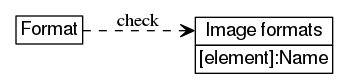The value is subject to the following constraints: image formats[the value]

This string does not correspond to an actual resource.

## Width

 number renderer/draw_plot/arguments: 2/ The width of the image

The value represents screen length, the standard unit of which is the pixel (px).

The value is subject to the following constraints: all of the following is true:

This number does not correspond to an actual resource.

## Height

 number renderer/draw_plot/arguments: 3/ The height of the image

The value represents screen length, the standard unit of which is the pixel (px).

The value is subject to the following constraints: all of the following is true:

This number does not correspond to an actual resource.

## Properties

 object renderer/draw_plot/arguments: 4/ Properties of the plot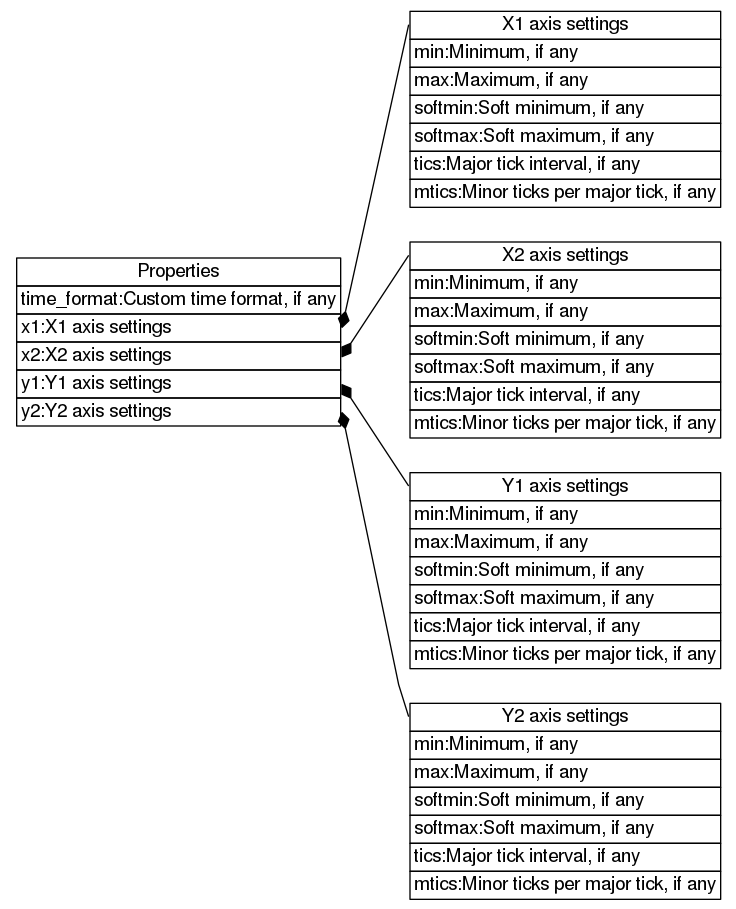This object does not correspond to an actual resource.

This object contains the following items:

## Lines

 array renderer/draw_plot/arguments: 5/ Plot data lines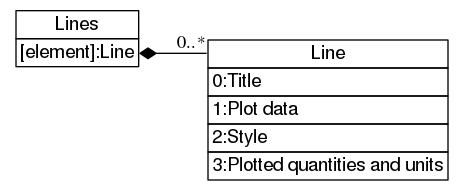This array does not correspond to an actual resource.

This array contains Line elements.

## Content type

 string renderer/draw_plot/results: 1/ The content type of the generated image

This string does not correspond to an actual resource.

## Image data

 string renderer/draw_plot/results: 2/ Generated image data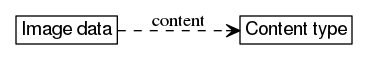The value contains content type data.

This string does not correspond to an actual resource.

# Properties: Custom time format, if any

 sum (optional string) renderer/draw_plot/arguments: 4/time_format/ Custom format to display time in, if any

This sum does not correspond to an actual resource.

This sum can assume the following values:

 constant null Default Use default time format string Custom time format Custom format to display time in

# Properties: X1 axis settings

 object renderer/draw_plot/arguments: 4/x1/ Configuration for X1 axis

This object does not correspond to an actual resource.

This object contains the following items:

## Minimum, if any

 sum (optional number) renderer/draw_plot/arguments: 4/x1/min/ Minimum value to display on the axis, if any

This sum does not correspond to an actual resource.

This sum can assume the following values:

 constant null Automatic The maximum value is derived from data and the soft maximum number Minimum Minimum value to display on the axis

## Maximum, if any

 sum (optional number) renderer/draw_plot/arguments: 4/x1/max/ Maximum value to display on the axis, if any

This sum does not correspond to an actual resource.

This sum can assume the following values:

 constant null Automatic The minimum value is derived from data and the soft minimum number Maximum Maximum value to display on the axis

## Soft minimum, if any

 sum (optional number) renderer/draw_plot/arguments: 4/x1/softmin/ Minimum value to display on the axis unless values exist below it, if any

This sum does not correspond to an actual resource.

This sum can assume the following values:

 constant null None No soft maximum defined number Soft minimum Minimum value to display on the axis unless values exist below it

## Soft maximum, if any

 sum (optional number) renderer/draw_plot/arguments: 4/x1/softmax/ Maximum value to display on the axis unless values exist above it, if any

This sum does not correspond to an actual resource.

This sum can assume the following values:

 constant null None No soft minimum defined number Soft maximum Maximum value to display on the axis unless values exist above it

## Major tick interval, if any

 sum (optional number) renderer/draw_plot/arguments: 4/x1/tics/ The interval, in value units, between major ticks of the axis, if any

This sum does not correspond to an actual resource.

This sum can assume the following values:

 constant null Automatic The tick interval is derived from data and size number Major tick interval The interval, in value units, between major ticks of the axis

## Minor ticks per major tick, if any

 sum (optional number) renderer/draw_plot/arguments: 4/x1/mtics/ The number of minor ticks between two major ticks, if any

This sum does not correspond to an actual resource.

This sum can assume the following values:

 constant null None There are no minor ticks number Minor ticks per major tick The number of minor ticks between two major ticks all of the following is true: the value is an integer the value is greater or equal to `0`

# Properties: X2 axis settings

 object renderer/draw_plot/arguments: 4/x2/ Configuration for X2 axis

This object does not correspond to an actual resource.

This object contains the following items:

## Minimum, if any

 sum (optional number) renderer/draw_plot/arguments: 4/x2/min/ Minimum value to display on the axis, if any

This sum does not correspond to an actual resource.

This sum can assume the following values:

 constant null Automatic The maximum value is derived from data and the soft maximum number Minimum Minimum value to display on the axis

## Maximum, if any

 sum (optional number) renderer/draw_plot/arguments: 4/x2/max/ Maximum value to display on the axis, if any

This sum does not correspond to an actual resource.

This sum can assume the following values:

 constant null Automatic The minimum value is derived from data and the soft minimum number Maximum Maximum value to display on the axis

## Soft minimum, if any

 sum (optional number) renderer/draw_plot/arguments: 4/x2/softmin/ Minimum value to display on the axis unless values exist below it, if any

This sum does not correspond to an actual resource.

This sum can assume the following values:

 constant null None No soft maximum defined number Soft minimum Minimum value to display on the axis unless values exist below it

## Soft maximum, if any

 sum (optional number) renderer/draw_plot/arguments: 4/x2/softmax/ Maximum value to display on the axis unless values exist above it, if any

This sum does not correspond to an actual resource.

This sum can assume the following values:

 constant null None No soft minimum defined number Soft maximum Maximum value to display on the axis unless values exist above it

## Major tick interval, if any

 sum (optional number) renderer/draw_plot/arguments: 4/x2/tics/ The interval, in value units, between major ticks of the axis, if any

This sum does not correspond to an actual resource.

This sum can assume the following values:

 constant null Automatic The tick interval is derived from data and size number Major tick interval The interval, in value units, between major ticks of the axis

## Minor ticks per major tick, if any

 sum (optional number) renderer/draw_plot/arguments: 4/x2/mtics/ The number of minor ticks between two major ticks, if any

This sum does not correspond to an actual resource.

This sum can assume the following values:

 constant null None There are no minor ticks number Minor ticks per major tick The number of minor ticks between two major ticks all of the following is true: the value is an integer the value is greater or equal to `0`

# Properties: Y1 axis settings

 object renderer/draw_plot/arguments: 4/y1/ Configuration for Y1 axis

This object does not correspond to an actual resource.

This object contains the following items:

## Minimum, if any

 sum (optional number) renderer/draw_plot/arguments: 4/y1/min/ Minimum value to display on the axis, if any

This sum does not correspond to an actual resource.

This sum can assume the following values:

 constant null Automatic The maximum value is derived from data and the soft maximum number Minimum Minimum value to display on the axis

## Maximum, if any

 sum (optional number) renderer/draw_plot/arguments: 4/y1/max/ Maximum value to display on the axis, if any

This sum does not correspond to an actual resource.

This sum can assume the following values:

 constant null Automatic The minimum value is derived from data and the soft minimum number Maximum Maximum value to display on the axis

## Soft minimum, if any

 sum (optional number) renderer/draw_plot/arguments: 4/y1/softmin/ Minimum value to display on the axis unless values exist below it, if any

This sum does not correspond to an actual resource.

This sum can assume the following values:

 constant null None No soft maximum defined number Soft minimum Minimum value to display on the axis unless values exist below it

## Soft maximum, if any

 sum (optional number) renderer/draw_plot/arguments: 4/y1/softmax/ Maximum value to display on the axis unless values exist above it, if any

This sum does not correspond to an actual resource.

This sum can assume the following values:

 constant null None No soft minimum defined number Soft maximum Maximum value to display on the axis unless values exist above it

## Major tick interval, if any

 sum (optional number) renderer/draw_plot/arguments: 4/y1/tics/ The interval, in value units, between major ticks of the axis, if any

This sum does not correspond to an actual resource.

This sum can assume the following values:

 constant null Automatic The tick interval is derived from data and size number Major tick interval The interval, in value units, between major ticks of the axis

## Minor ticks per major tick, if any

 sum (optional number) renderer/draw_plot/arguments: 4/y1/mtics/ The number of minor ticks between two major ticks, if any

This sum does not correspond to an actual resource.

This sum can assume the following values:

 constant null None There are no minor ticks number Minor ticks per major tick The number of minor ticks between two major ticks all of the following is true: the value is an integer the value is greater or equal to `0`

# Properties: Y2 axis settings

 object renderer/draw_plot/arguments: 4/y2/ Configuration for Y2 axis

This object does not correspond to an actual resource.

This object contains the following items:

## Minimum, if any

 sum (optional number) renderer/draw_plot/arguments: 4/y2/min/ Minimum value to display on the axis, if any

This sum does not correspond to an actual resource.

This sum can assume the following values:

 constant null Automatic The maximum value is derived from data and the soft maximum number Minimum Minimum value to display on the axis

## Maximum, if any

 sum (optional number) renderer/draw_plot/arguments: 4/y2/max/ Maximum value to display on the axis, if any

This sum does not correspond to an actual resource.

This sum can assume the following values:

 constant null Automatic The minimum value is derived from data and the soft minimum number Maximum Maximum value to display on the axis

## Soft minimum, if any

 sum (optional number) renderer/draw_plot/arguments: 4/y2/softmin/ Minimum value to display on the axis unless values exist below it, if any

This sum does not correspond to an actual resource.

This sum can assume the following values:

 constant null None No soft maximum defined number Soft minimum Minimum value to display on the axis unless values exist below it

## Soft maximum, if any

 sum (optional number) renderer/draw_plot/arguments: 4/y2/softmax/ Maximum value to display on the axis unless values exist above it, if any

This sum does not correspond to an actual resource.

This sum can assume the following values:

 constant null None No soft minimum defined number Soft maximum Maximum value to display on the axis unless values exist above it

## Major tick interval, if any

 sum (optional number) renderer/draw_plot/arguments: 4/y2/tics/ The interval, in value units, between major ticks of the axis, if any

This sum does not correspond to an actual resource.

This sum can assume the following values:

 constant null Automatic The tick interval is derived from data and size number Major tick interval The interval, in value units, between major ticks of the axis

## Minor ticks per major tick, if any

 sum (optional number) renderer/draw_plot/arguments: 4/y2/mtics/ The number of minor ticks between two major ticks, if any

This sum does not correspond to an actual resource.

This sum can assume the following values:

 constant null None There are no minor ticks number Minor ticks per major tick The number of minor ticks between two major ticks all of the following is true: the value is an integer the value is greater or equal to `0`

# Plot data: Plot point

 tuple renderer/draw_plot/arguments: 5/N/2/N/ Point to plot

This tuple does not correspond to an actual resource.

This tuple contains the following items:

 1 X 2 Y, if any

## X

 number renderer/draw_plot/arguments: 5/N/2/N/1/ X coordinate to plot

This number does not correspond to an actual resource.

## Y, if any

 sum (variant) renderer/draw_plot/arguments: 5/N/2/N/2/ Y coordinate to plot, if any

This sum does not correspond to an actual resource.

This sum can assume the following values:

 constant false None Data for this X position is absent number Y Y coordinate to plot

# Plotted quantities and units: X axis quantity and unit

 object renderer/draw_plot/arguments: 5/N/4/1/ The quantity and unit for X axis

This object does not correspond to an actual resource.

This object contains the following items:

## X axis quantity

 string renderer/draw_plot/arguments: 5/N/4/1/quantity/ The quantity for X axis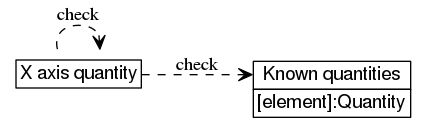The value is subject to the following constraints: known quantities[the value]

This string does not correspond to an actual resource.

## X axis unit

 string renderer/draw_plot/arguments: 5/N/4/1/unit/ The unit for X axis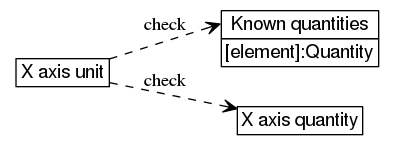The value is subject to the following constraints: known quantities[X axis quantity].units[the value]

This string does not correspond to an actual resource.

# Plotted quantities and units: Y axis quantity and unit

 object renderer/draw_plot/arguments: 5/N/4/2/ The quantity and unit for Y axis

This object does not correspond to an actual resource.

This object contains the following items:

## Y axis quantity

 string renderer/draw_plot/arguments: 5/N/4/2/quantity/ The quantity for Y axis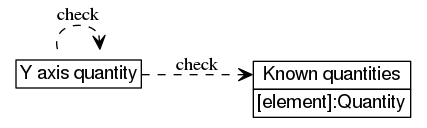The value is subject to the following constraints: known quantities[the value]

This string does not correspond to an actual resource.

## Y axis unit

 string renderer/draw_plot/arguments: 5/N/4/2/unit/ The unit for Y axis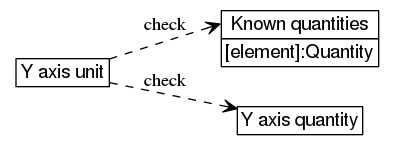The value is subject to the following constraints: known quantities[Y axis quantity].units[the value]

This string does not correspond to an actual resource.

# Lines: Line

 tuple renderer/draw_plot/arguments: 5/N/ Plot data line settings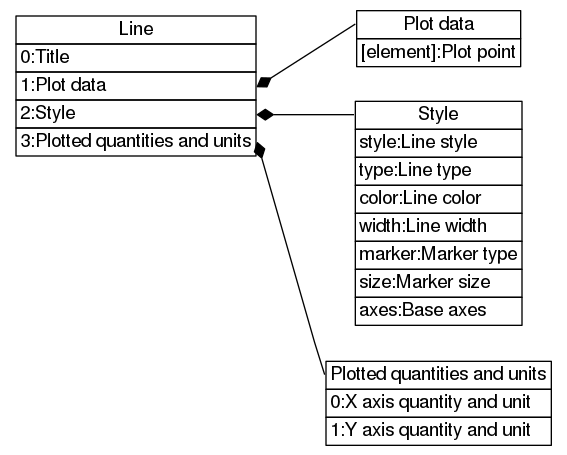This tuple does not correspond to an actual resource.

This tuple contains the following items:

 1 Title 2 Plot data 3 Style 4 Plotted quantities and units

## Title

 string renderer/draw_plot/arguments: 5/N/1/ Plot line title

This string does not correspond to an actual resource.

## Plot data

 array renderer/draw_plot/arguments: 5/N/2/ Data to plot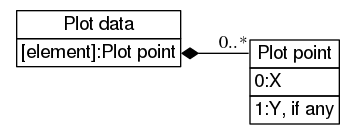This array does not correspond to an actual resource.

This array contains Plot point elements.

## Style

 object renderer/draw_plot/arguments: 5/N/3/ Style of the plot line

This object does not correspond to an actual resource.

This object contains the following items:

### Line style

 string renderer/draw_plot/arguments: 5/N/3/style/ The style of the line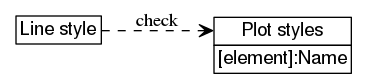The value is subject to the following constraints: plot styles[the value]

This string does not correspond to an actual resource.

### Line type

 string renderer/draw_plot/arguments: 5/N/3/type/ The type of the line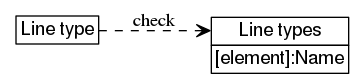The value is subject to the following constraints: line types[the value]

This string does not correspond to an actual resource.

### Line color

 string renderer/draw_plot/arguments: 5/N/3/color/ The color of the line

The value is subject to the following constraints: the value matches ^[0-9A-F]{6}\$

This string does not correspond to an actual resource.

### Line width

 number renderer/draw_plot/arguments: 5/N/3/width/ The width of the line

The value represents screen length, the standard unit of which is the pixel (px).

The value is subject to the following constraints: all of the following is true:

This number does not correspond to an actual resource.

### Marker type

 string renderer/draw_plot/arguments: 5/N/3/marker/ The type of the marker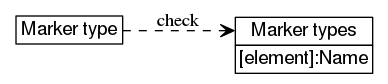The value is subject to the following constraints: marker types[the value]

This string does not correspond to an actual resource.

### Marker size

 number renderer/draw_plot/arguments: 5/N/3/size/ The size of the markers

The value represents screen length, the standard unit of which is the pixel (px).

The value is subject to the following constraints: all of the following is true:

This number does not correspond to an actual resource.

### Base axes

 string renderer/draw_plot/arguments: 5/N/3/axes/ The axes on which the line should be plotted. Units of lines plotted on the same axes must match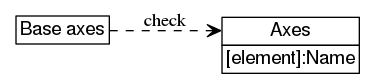The value is subject to the following constraints: axes[the value]

This string does not correspond to an actual resource.

## Plotted quantities and units

 tuple renderer/draw_plot/arguments: 5/N/4/ Quantities and units to be plotted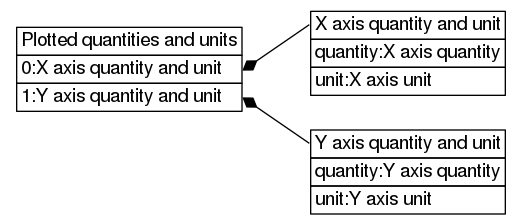This tuple does not correspond to an actual resource.

This tuple contains the following items:

# Draw a pie meter

 call renderer/draw_pie_meter/ Generate a multi-sector meter image with an arrow indicating value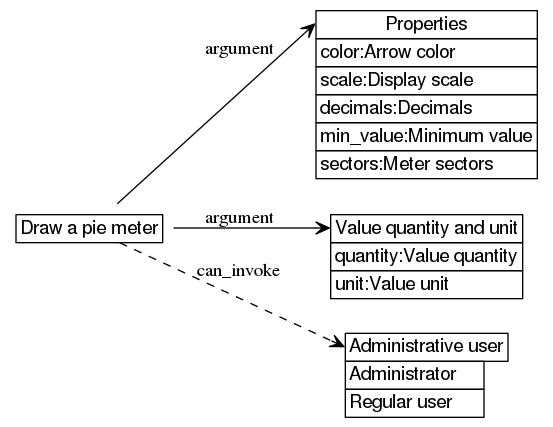Performing the function call is denied if not administrative user

The call accepts the following arguments:

1. Format: string
2. Width: number
3. Height: number
4. Properties: object "Properties"
5. Value: number
6. Value quantity and unit: object "Value quantity and unit"

The call returns the following results:

1. Content type: string
2. Image data: string

Sample CLI invocation:

```uom invoke "renderer/draw_pie_meter" "\"png\"" "768" "5120" "{\"decimals\":0,\"scale\":\"linear\",\"sectors\":[{\"value\":73,\"name\":\"Too low\",\"color\":\"B40977\"},{\"value\":92,\"name\":\"Low\",\"color\":\"133436\"},{\"value\":117,\"name\":\"Average\",\"color\":\"DA3EC5\"},{\"value\":133,\"name\":\"High\",\"color\":\"637E24\"},{\"value\":163,\"name\":\"Too high\",\"color\":\"808072\"}],\"min_value\":49,\"color\":\"AB84E6\"}" "53"
```

```"<binary data of type image/jpeg>"
```

Sample `requests` invocation:

```print(requests.post('http://192.168.0.100/restapi/renderer/draw_pie_meter/',auth=auth,headers=headers,json=['jpeg', 1280, 64, {'decimals': 0, 'scale': 'log', 'sectors': [{'value': 70, 'name': 'Low', 'color': '9336D2'}, {'value': 76, 'name': 'High', 'color': 'FD448E'}], 'min_value': 67, 'color': '38C3C9'}, 70]).json())
```

```'<binary data of type image/jpeg>'
```

## Format

 string renderer/draw_pie_meter/arguments: 1/ Image formatThe value is subject to the following constraints: image formats[the value]

This string does not correspond to an actual resource.

## Width

 number renderer/draw_pie_meter/arguments: 2/ The width of the image

The value represents screen length, the standard unit of which is the pixel (px).

The value is subject to the following constraints: all of the following is true:

This number does not correspond to an actual resource.

## Height

 number renderer/draw_pie_meter/arguments: 3/ The height of the image

The value represents screen length, the standard unit of which is the pixel (px).

The value is subject to the following constraints: all of the following is true:

This number does not correspond to an actual resource.

## Properties

 object renderer/draw_pie_meter/arguments: 4/ Properties of the meter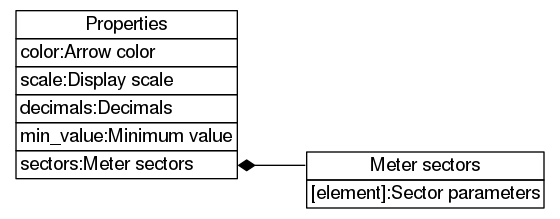This object does not correspond to an actual resource.

This object contains the following items:

## Value

 number renderer/draw_pie_meter/arguments: 5/ Meter value to display

This number does not correspond to an actual resource.

## Content type

 string renderer/draw_pie_meter/results: 1/ The content type of the generated image

This string does not correspond to an actual resource.

## Image data

 string renderer/draw_pie_meter/results: 2/ Generated image dataThe value contains content type data.

This string does not correspond to an actual resource.

# Properties: Arrow color

 string renderer/draw_pie_meter/arguments: 4/color/ The color of the meter arrow

The value is subject to the following constraints: the value matches ^[0-9A-F]{6}\$

This string does not correspond to an actual resource.

# Properties: Display scale

 string renderer/draw_pie_meter/arguments: 4/scale/ The scale function to use for display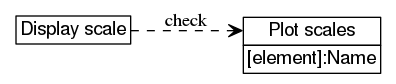The value is subject to the following constraints: plot scales[the value]

This string does not correspond to an actual resource.

# Properties: Decimals

 number renderer/draw_pie_meter/arguments: 4/decimals/ Number of decimal places to show in displayed value

The value represents count, the standard unit of which is the time (times).

The value is subject to the following constraints: all of the following is true:

This number does not correspond to an actual resource.

# Properties: Minimum value

 number renderer/draw_pie_meter/arguments: 4/min_value/ The minimum value to be displayed on the meter

This number does not correspond to an actual resource.

# Properties: Meter sectors

 array renderer/draw_pie_meter/arguments: 4/sectors/ Parameters of meter sectors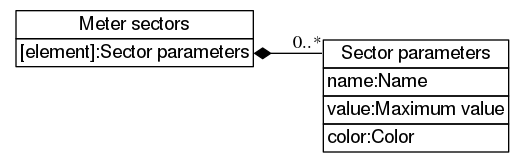This array does not correspond to an actual resource.

This array contains Sector parameters elements.

## Sector parameters

 object renderer/draw_pie_meter/arguments: 4/sectors/N/ Parameters of a meter sector

This object does not correspond to an actual resource.

This object contains the following items:

### Name

 string renderer/draw_pie_meter/arguments: 4/sectors/N/name/ The name of the sector

This string does not correspond to an actual resource.

### Maximum value

 number renderer/draw_pie_meter/arguments: 4/sectors/N/value/ The maximum value for the sector

This number does not correspond to an actual resource.

### Color

 string renderer/draw_pie_meter/arguments: 4/sectors/N/color/ The color of the sector

The value is subject to the following constraints: the value matches ^[0-9A-F]{6}\$

This string does not correspond to an actual resource.

# Draw a pie meter: Value quantity and unit

 object renderer/draw_pie_meter/arguments: 6/ The quantity and unit for value

This object does not correspond to an actual resource.

This object contains the following items:

## Value quantity

 string renderer/draw_pie_meter/arguments: 6/quantity/ The quantity for value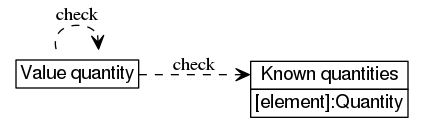The value is subject to the following constraints: known quantities[the value]

This string does not correspond to an actual resource.

## Value unit

 string renderer/draw_pie_meter/arguments: 6/unit/ The unit for value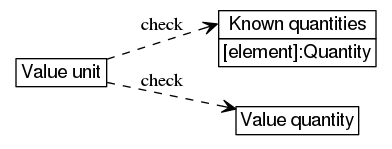The value is subject to the following constraints: known quantities[value quantity].units[the value]

This string does not correspond to an actual resource.

# Draw a message

 call renderer/draw_message/ Generate an image with a message in its center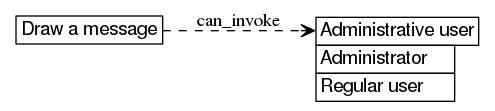Performing the function call is denied if not administrative user

The call accepts the following arguments:

1. Format: string
2. Width: number
3. Height: number
4. Message: string

The call returns the following results:

1. Content type: string
2. Image data: string

Sample JS `request` invocation:

```request.post({url:"renderer/draw_message/",body:["gif",7680,128,"Message!"]});
```

```"<binary data of type image/jpeg>"
```

Sample `curl` invocation:

```curl --digest -u admin:1234 -H "X-CSRF: x" -H "Content-type: application/json" -H "Accept: application/json" --data-binary "[\"jpeg\",15360,256,\"Message!\"]" "http://192.168.0.100/restapi/renderer/draw_message/"
```

```"<binary data of type image/jpeg>"
```

## Format

 string renderer/draw_message/arguments: 1/ Image formatThe value is subject to the following constraints: image formats[the value]

This string does not correspond to an actual resource.

## Width

 number renderer/draw_message/arguments: 2/ The width of the image

The value represents screen length, the standard unit of which is the pixel (px).

The value is subject to the following constraints: all of the following is true:

This number does not correspond to an actual resource.

## Height

 number renderer/draw_message/arguments: 3/ The height of the image

The value represents screen length, the standard unit of which is the pixel (px).

The value is subject to the following constraints: all of the following is true:

This number does not correspond to an actual resource.

## Message

 string renderer/draw_message/arguments: 4/ Message text

This string does not correspond to an actual resource.

## Content type

 string renderer/draw_message/results: 1/ The content type of the generated image

This string does not correspond to an actual resource.

## Image data

 string renderer/draw_message/results: 2/ Generated image dataThe value contains content type data.

This string does not correspond to an actual resource.

# Plot styles

 map renderer/known_plot_styles/ Known plot styles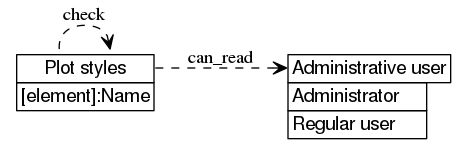Retrieving the value is denied if not administrative user

This map does not support direct modification.

This map contains Name elements.

Creating elements in this collection is not supported.

Sample `curl` read command:

```curl --digest -u admin:1234 -H "Accept: application/json" "http://192.168.0.100/restapi/renderer/known_plot_styles/"
```

```uom get "renderer/known_plot_styles"
```

## Name

 string renderer/known_plot_styles/S/ Name of plot style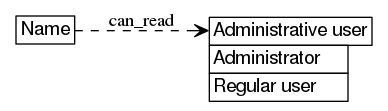Retrieving the value is denied if not administrative user

This string does not support direct modification.

Sample JS `request` read command (note that `_name` is a variable):

```request.get({url:"renderer/known_plot_styles/"+_name+"/"},function(error,response,body) {console.log(body)});
```

```"Bezier smoothed lines"
```

Sample CLI read command (note that `name` is a variable):

```uom get "renderer/known_plot_styles/\${name}"
```

```"Lines only"
```

# Plot scales

 map renderer/known_scales/ Known plot scales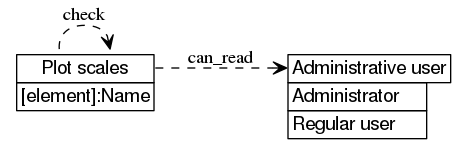Retrieving the value is denied if not administrative user

This map does not support direct modification.

This map contains Name elements.

Creating elements in this collection is not supported.

Sample `uom` library read command:

```print(uom.dump(uom.renderer.known_scales))
```

```{linear="linear",log="logarithmic"}
```

Sample `requests` read command:

```print(requests.get('http://192.168.0.100/restapi/renderer/known_scales/',auth=auth,headers=headers).json())
```

```{'linear': 'linear', 'log': 'logarithmic'}
```

## Name

 string renderer/known_scales/S/ Name of plot scaleRetrieving the value is denied if not administrative user

This string does not support direct modification.

Sample `requests` read command (note that `_name` is a variable):

```print(requests.get('http://192.168.0.100/restapi/renderer/known_scales/'+_name+'/',auth=auth,headers=headers).json())
```

```'linear'
```

Sample `curl` read command (note that `:name` is a URL template argument):

```curl --digest -u admin:1234 -H "Accept: application/json" "http://192.168.0.100/restapi/renderer/known_scales/:name/"
```

```"logarithmic"
```

# Line types

 map renderer/known_line_types/ Known line types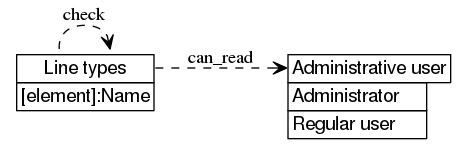Retrieving the value is denied if not administrative user

This map does not support direct modification.

This map contains Name elements.

Creating elements in this collection is not supported.

Sample JS `request` read command:

```request.get({url:"renderer/known_line_types/"},function(error,response,body) {console.log(body)});
```

```{"dashed":"Dashed","dotted":"Dotted","solid":"Solid"}
```

Sample `curl` read command:

```curl --digest -u admin:1234 -H "Accept: application/json" "http://192.168.0.100/restapi/renderer/known_line_types/"
```

```{"dashed":"Dashed","dotted":"Dotted","solid":"Solid"}
```

## Name

 string renderer/known_line_types/S/ Name of line typeRetrieving the value is denied if not administrative user

This string does not support direct modification.

Sample `requests` read command (note that `_name` is a variable):

```print(requests.get('http://192.168.0.100/restapi/renderer/known_line_types/'+_name+'/',auth=auth,headers=headers).json())
```

```'Solid'
```

Sample CLI read command (note that `name` is a variable):

```uom get "renderer/known_line_types/\${name}"
```

```"Dashed"
```

# Marker types

 map renderer/known_marker_types/ Known marker types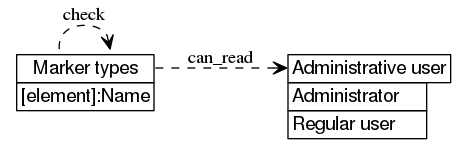Retrieving the value is denied if not administrative user

This map does not support direct modification.

This map contains Name elements.

Creating elements in this collection is not supported.

Sample `requests` read command:

```print(requests.get('http://192.168.0.100/restapi/renderer/known_marker_types/',auth=auth,headers=headers).json())
```

Sample `curl` read command:

```curl --digest -u admin:1234 -H "Accept: application/json" "http://192.168.0.100/restapi/renderer/known_marker_types/"
```

## Name

 string renderer/known_marker_types/S/ Name of marker typeRetrieving the value is denied if not administrative user

This string does not support direct modification.

Sample JS `request` read command (note that `_name` is a variable):

```request.get({url:"renderer/known_marker_types/"+_name+"/"},function(error,response,body) {console.log(body)});
```

```"Dot"
```

Sample `uom` library read command (note that `_name` is a variable):

```print(uom.dump(uom.renderer.known_marker_types[_name]))
```

```"Filled triangle"
```

# Axes

 map renderer/known_axes/ Known axis combinations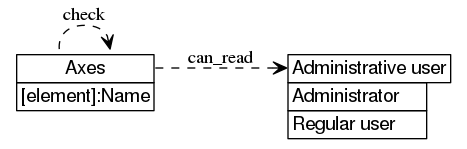Retrieving the value is denied if not administrative user

This map does not support direct modification.

This map contains Name elements.

Creating elements in this collection is not supported.

Sample JS `request` read command:

```request.get({url:"renderer/known_axes/"},function(error,response,body) {console.log(body)});
```

```{"x2y2":"X2, Y2","x2y1":"X2, Y1","x1y1":"X1, Y1","x1y2":"X1, Y2"}
```

Sample `curl` read command:

```curl --digest -u admin:1234 -H "Accept: application/json" "http://192.168.0.100/restapi/renderer/known_axes/"
```

```{"x2y2":"X2, Y2","x2y1":"X2, Y1","x1y1":"X1, Y1","x1y2":"X1, Y2"}
```

## Name

 string renderer/known_axes/S/ Name of axis combinationRetrieving the value is denied if not administrative user

This string does not support direct modification.

Sample `requests` read command (note that `_name` is a variable):

```print(requests.get('http://192.168.0.100/restapi/renderer/known_axes/'+_name+'/',auth=auth,headers=headers).json())
```

```'X2, Y1'
```

Sample CLI read command (note that `name` is a variable):

```uom get "renderer/known_axes/\${name}"
```

```"X1, Y1"
```

# Image formats

 map renderer/known_image_formats/ Known image formats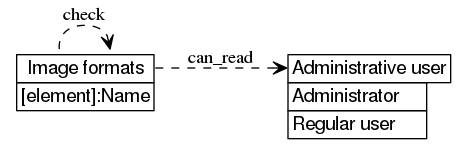Retrieving the value is denied if not administrative user

This map does not support direct modification.

This map contains Name elements.

Creating elements in this collection is not supported.

Sample `uom` library read command:

```print(uom.dump(uom.renderer.known_image_formats))
```

```{gif="GIF",jpeg="JPEG",png="PNG",svg="SVG"}
```

```uom get "renderer/known_image_formats"
```

```{"svg":"SVG","gif":"GIF","jpeg":"JPEG","png":"PNG"}
```

## Name

 string renderer/known_image_formats/S/ Name of image formatRetrieving the value is denied if not administrative user

This string does not support direct modification.

Sample CLI read command (note that `name` is a variable):

```uom get "renderer/known_image_formats/\${name}"
```

```"PNG"
```

Sample `curl` read command (note that `:name` is a URL template argument):

```curl --digest -u admin:1234 -H "Accept: application/json" "http://192.168.0.100/restapi/renderer/known_image_formats/:name/"
```

```"GIF"
```